# 大模型訓練難於上青天？效率超群、易用的“李白”模型庫來了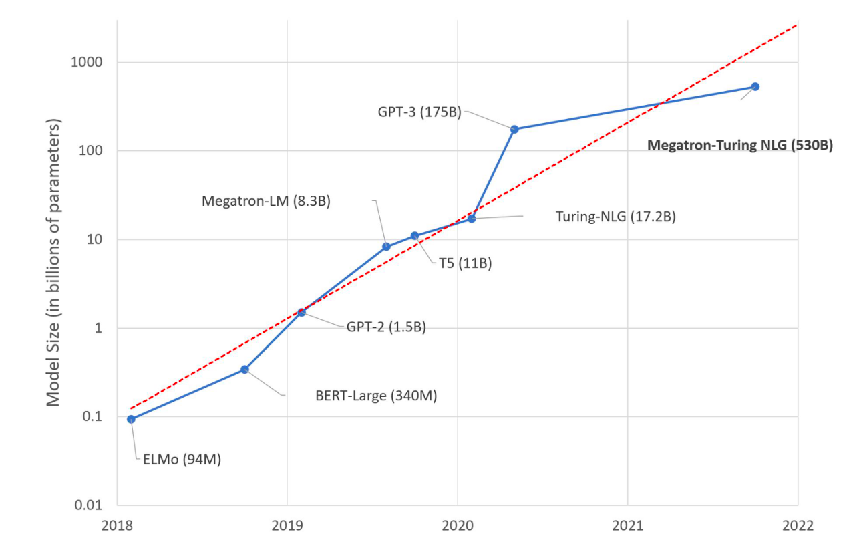LiBai 模型庫地址：\ https://github.com/Oneflow-Inc/libai

1

# 一鍵自動分散式訓練，效能超越 Megatron-LM 和 DeepSpeed

• 支援單卡程式碼平滑地擴充套件到分散式。LiBai 內建的模型與 PyTorch 保持一致風格，大大降低學習和使用成本，只需要簡單配置，就可以便捷地擴充套件至任意規模的並行。這意味著，你可以在單卡上增加新功能，進行模型除錯，跑通程式碼後再絲滑地遷移到分散式上進行訓練。如果完全不想配置分散式訓練，或是覺得手動配置的分散式訓練太慢，那可以試用分散式託管特性，只需安裝自動並行的包（https://libai.readthedocs.io/en/latest/tutorials/basics/Auto_Parallel.html ），並在 LiBai 裡配置一行 graph.auto_parallel=True，就可以專注於模型本身，在完全不用操心分散式的同時獲得較快的訓練速度。
• 相容 Hugging Face。OneFlow 和 PyTorch 在 API 層次高度相容，可以通過簡單的程式碼修改就可以匯入 Hugging Face 模型，只須 import oneflow as torch ，基於 LiBai 的資料並行、自動混合精度、Activation Checkpoint、ZeRO 等機制進行一個大規模模型的訓練。如果把模型的個別層次替換為 LiBai 內建的 layers ，就可以使用 3D 並行來訓練一個大模型。
• 模組化設計。在 LiBai 的實現中，不僅為模型構建提供可複用的基礎計算模組，也針對資料載入、訓練邏輯、指標計算等做了抽象和模組化處理，方便使用者根據自己的需求重寫，然後作為外掛整合到 LiBai 的訓練系統中進行訓練。
• 開箱即用。大模型訓練通常需要依賴一些技術，LiBai 提供了混合精度訓練、梯度重計算、梯度累加、ZeRO 等特性，可以輕鬆與資料並行、模型並行、流水並行組合使用。
• 快速復現實驗。OneFlow 團隊參考了 Detectron2 LazyConfig（https://github.com/facebookresearch/detectron2/blob/main/docs/tutorials/lazyconfigs.md ） 來構建 LiBai 的配置系統，相比於傳統的 argparse 和 yacs-based 配置方式，LiBai 的配置系統更加靈活，使用 Python 語法完成整體構建，所以新增新的引數和模組非常方便，只需要 import 對應的模組即可完成新模組的新增。同時，訓練配置還可以序列化成 yaml 檔案進行儲存，方便直接在檔案中進行關鍵字搜尋來查詢配置項，如果使用者想要復現之前的實驗的結果，也直接傳入儲存的 config.yaml 作為訓練配置，保留非常多指令碼的檔案既不利於檢視有效修改，在復現實驗的同時也容易弄混實驗配置。
• 高效效能。通過和 Megatron-LM 進行嚴格的 kernel 對齊，實現了多種 kernel fusion 操作，同時得益於 OneFlow 靜態圖的設計，不管是單卡效能還是各種組合並行的效率，LiBai 都優於英偉達深度優化的 Megatron-LM 和微軟的 DeepSpeed。

• Megatron-LM 固定 commit：https://github.com/NVIDIA/Megatron-LM/commit/e156d2fea7fc5c98e645f7742eb86b643956d840
• LiBai commit: https://github.com/Oneflow-Inc/libai/commit/9fc504c457da4fd1e92d854c60b7271c89a55222
• OneFlow commit: https://github.com/Oneflow-Inc/oneflow/commit/55b822e4d3c88757d11077d7546981309125c73f

DP 資料並行、MP 模型並行、PP 流水並行、2D 並行、3D 並行

fp16：開啟混合精度訓練 (amp)

nl: num layers (當 Pipeline parallel size = 8 時，為了讓每個 stage 有相對數量的 layer 進行計算，我們將 num layers 從 24 調整為 48)

ac: enable activation checkpointing mb: micro-batch size per gpu gb: global batch size total dxmxp，其中: d = 資料並行度(data-parallel-size) m = 模型並行度(\ tensor-model-parallel-size) p = 流水並行度(\ pipeline-model-parallel-size)

1n1g 表示單機單卡，1n8g 表示單機 8 卡, 2n8g 表示 2 機每機 8 卡共 16 卡， 4n8g 表示 4 機共 32 卡 grad_acc_num_step = global_batch_size / (micro_batch_size * data_parallel_size) 展示的結果為 Throughout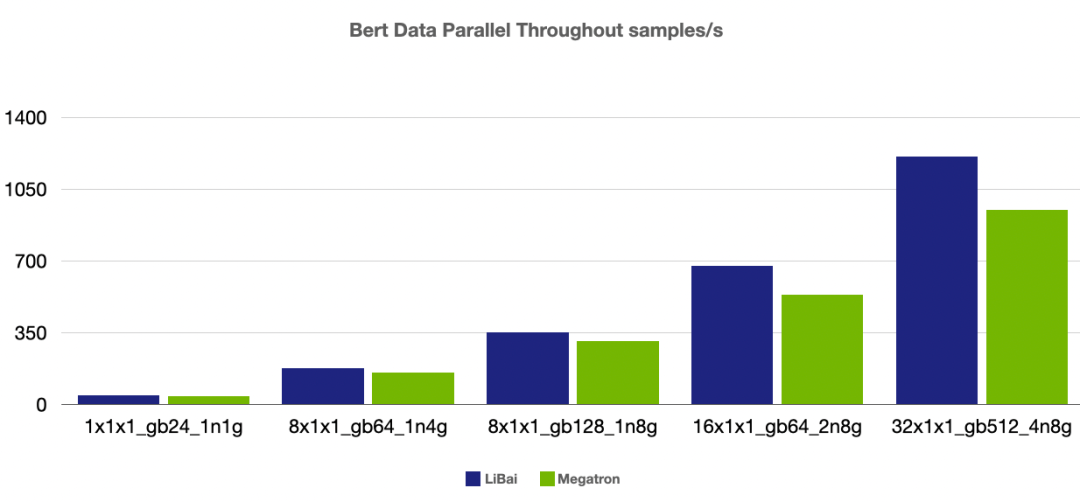（注：本組 num layers = 24，開啟 amp，1n1g micro-batch size = 24, 其餘組 micro-batch size = 16）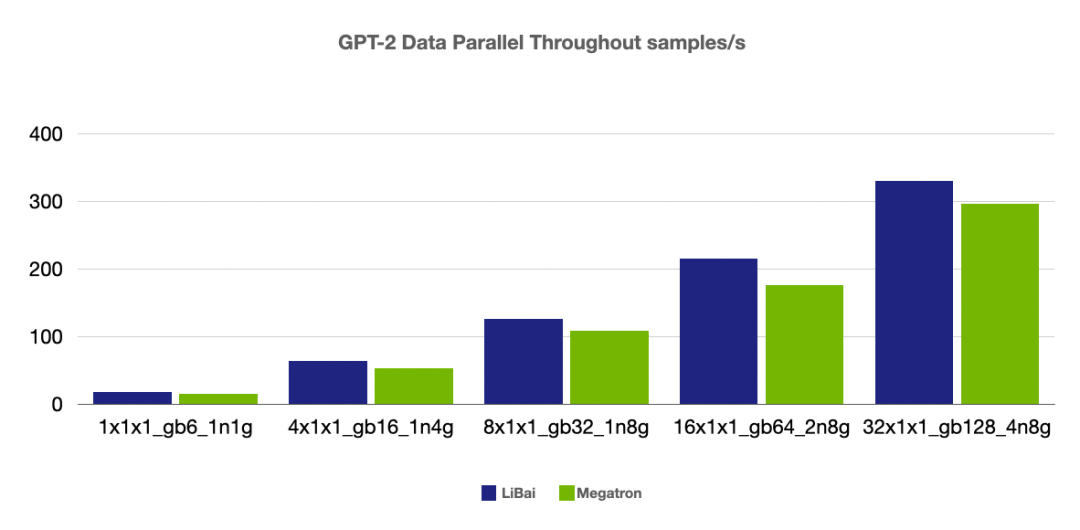（注：本組 num layers = 24，開啟 amp，1n1g micro-batch size = 6, 其餘組 micro-batch size = 4）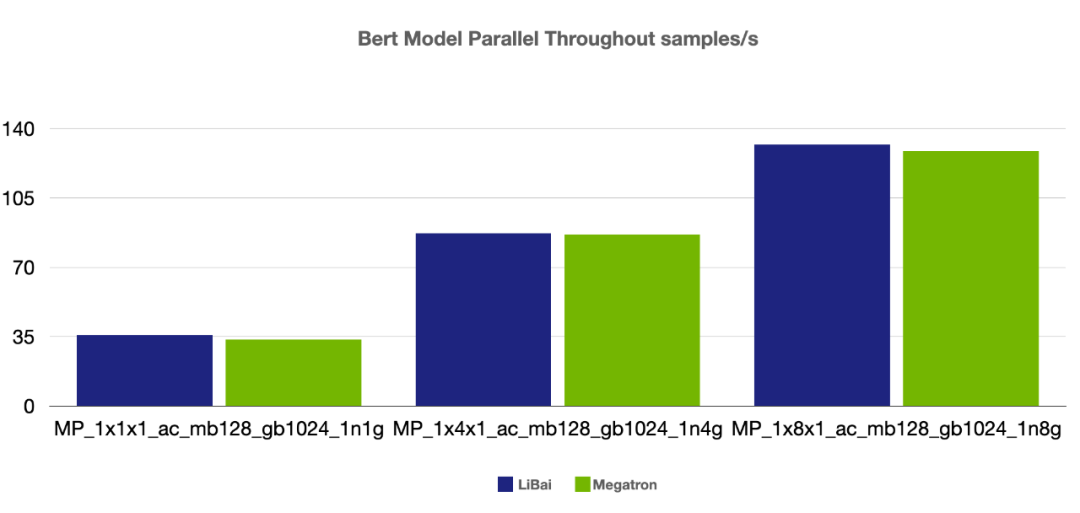（注：本組 num layers = 24，開啟 amp， 開啟 activation checkpointing，

micro-batch size = 128， global batch size = 1024， grad acc step = 8）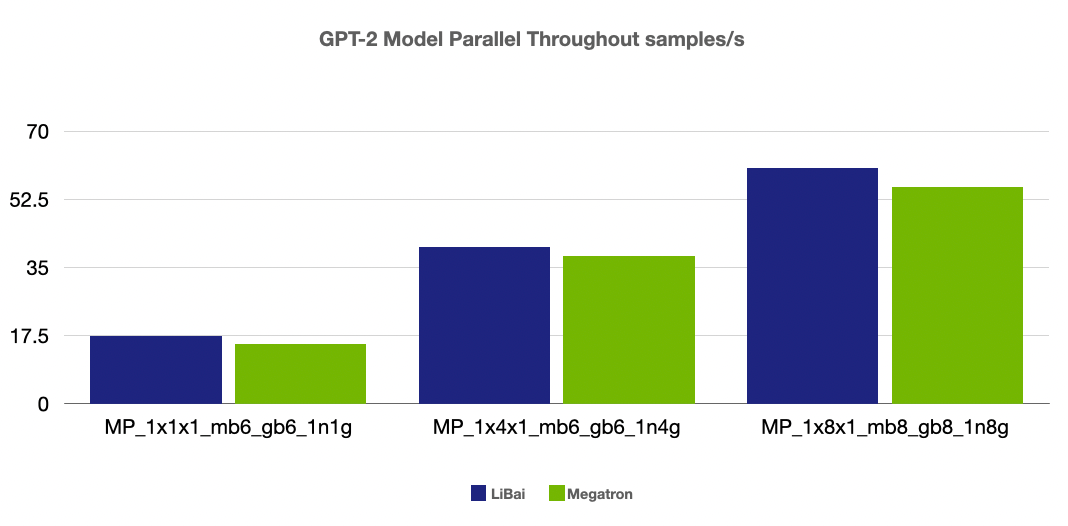（注：本組 num layers = 24，開啟 amp）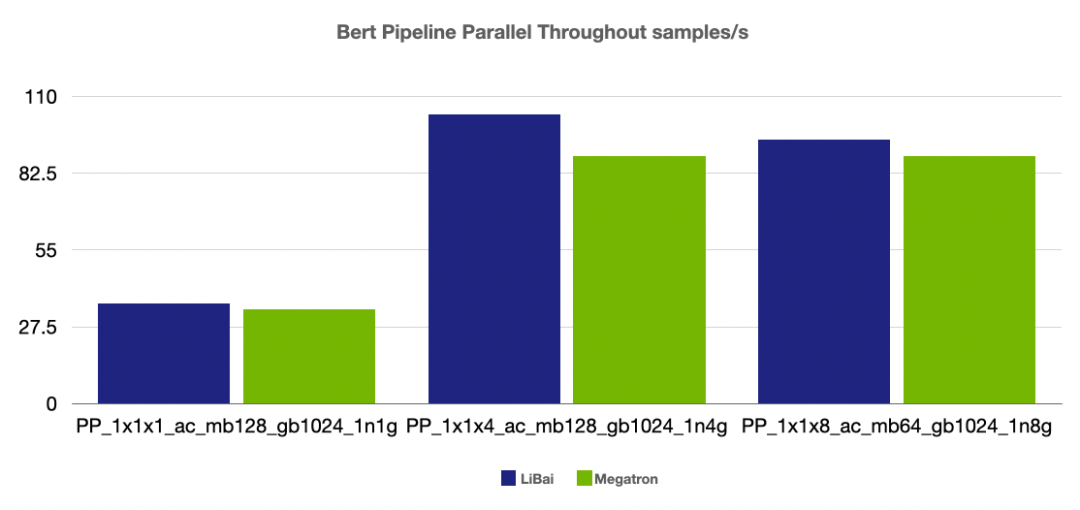（注：前兩組 num layers = 24，grad acc step = 8， 最後一組 num layers = 48, grad acc step = 16，均開啟 amp，開啟 activation checkpointing）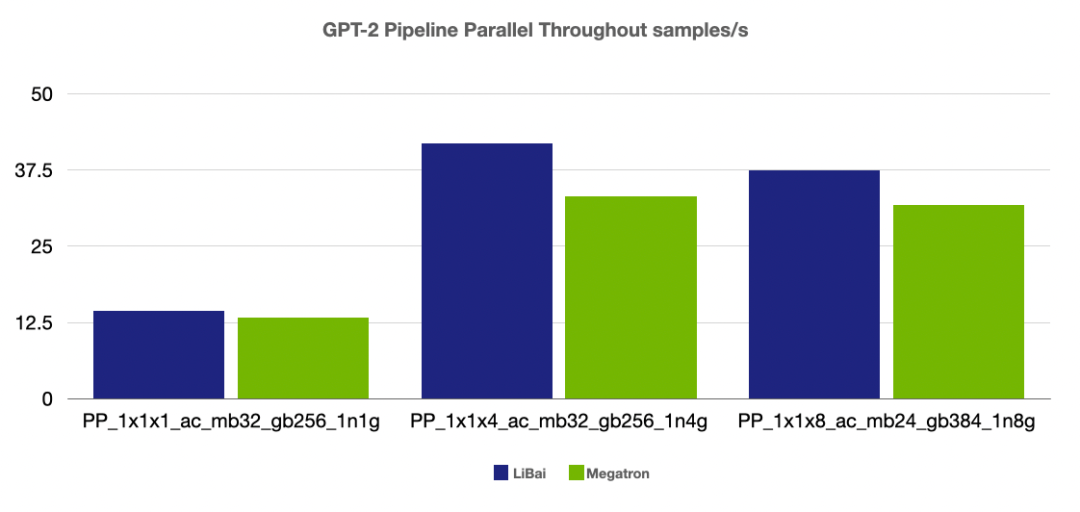（注：前兩組 num layers = 24，grad acc step = 8， 最後一組 num layers = 48, grad acc step = 16，均開啟 amp，開啟 activation checkpointing）

2-D 並行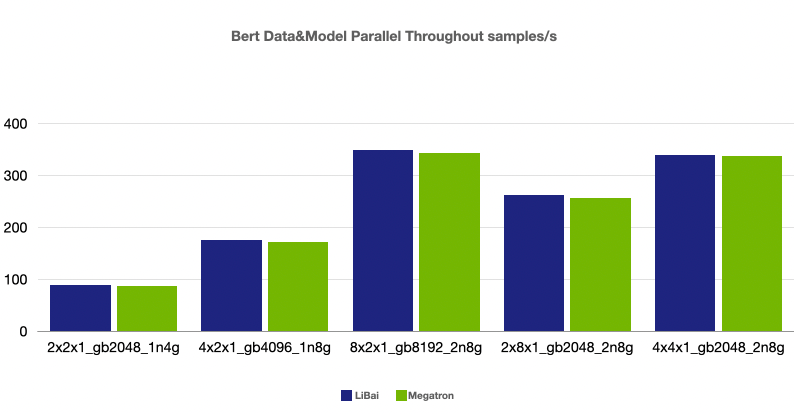（注：本組均為 num layers = 24，均開啟 amp，開啟 activation checkpointing， micro-batch size = 128，grad acc step = 8）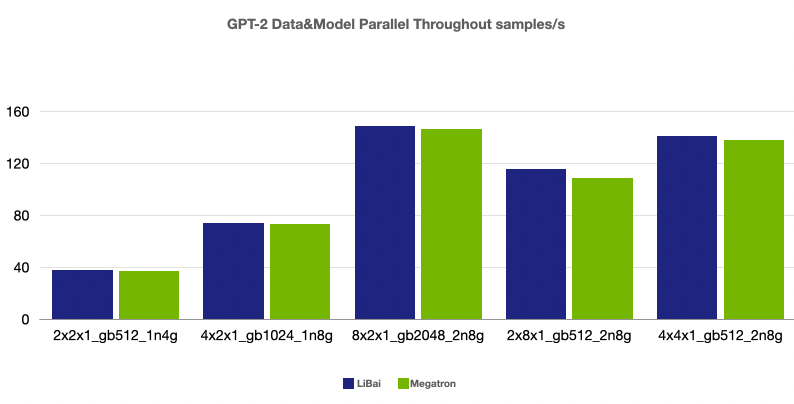（注：本組均為 num layers = 24，均開啟 amp，開啟 activation checkpointing， micro-batch size = 32，grad acc step = 8）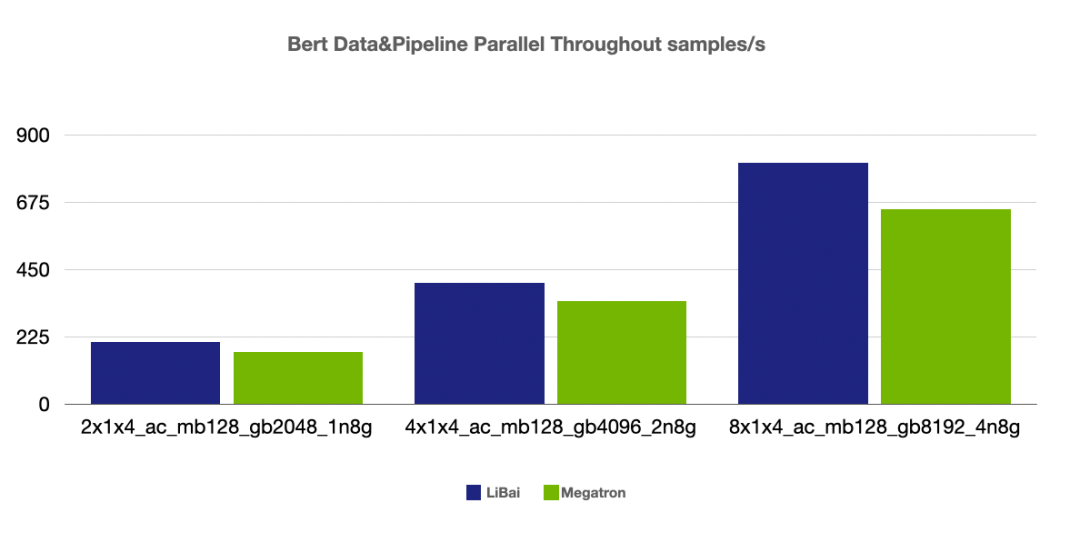（注：本組均為 num layers = 24，均開啟 amp，開啟 activation checkpointing， micro-batch size = 128，grad acc step = 8）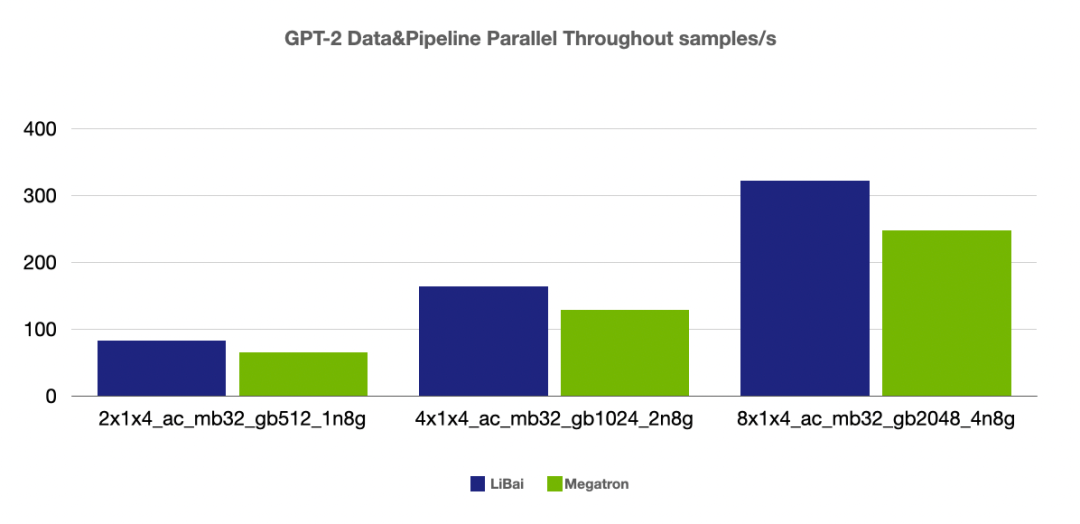（注：本組均為 num layers = 24，均開啟 amp，開啟 activation checkpointing， micro-batch size = 32，grad acc step = 8）

3-D 並行（注：本組均為 num layers = 24，均開啟 amp，開啟 activation checkpointing，grad acc step = 8）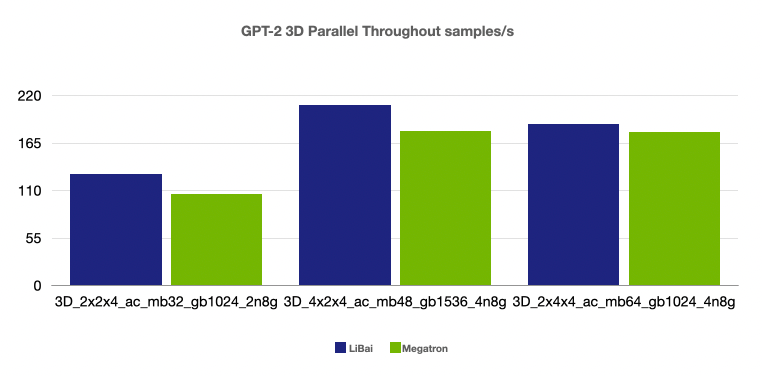（注：本組均為 num layers = 24，均開啟 amp，開啟 activation checkpointing，grad acc step = 8）

2

# 人有我優，人無我有：LiBai vs 其他訓練方案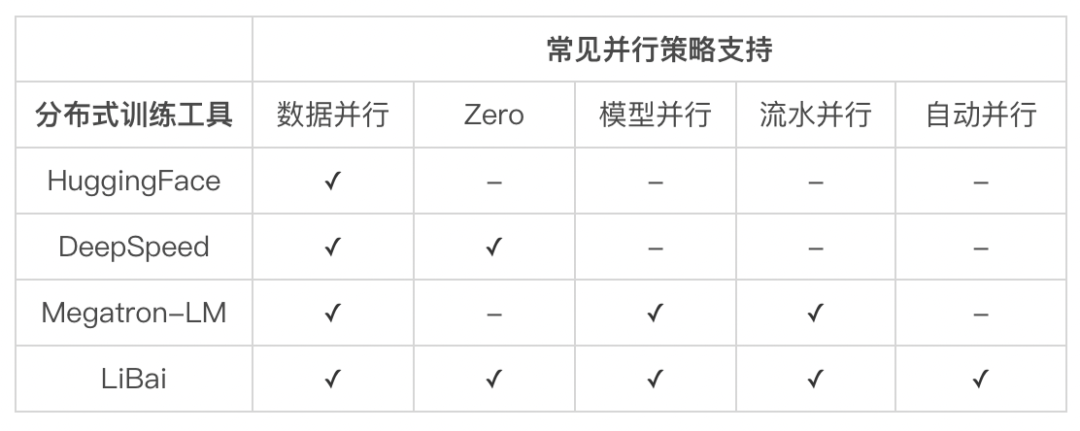HuggingFace：提供了全面的 SOTA Transformer 模型 ，便於使用預訓練好的模型進行微調，提供強大的社群和生態，方便開發者使用預訓練好的模型。但它只支援資料並行的方式，如果模型超過單個 GPU 視訊記憶體容量就不適用了，而且想用它做從零開始訓練速度也比較受限。

FairSeq：主要針對序列模型，在 NLP 和 CV 大一統的趨勢下，缺少對 CV 模型的支援。

Megatron-LM：基於 PyTorch 實現了資料並行、模型並行和流水並行，效能高，可以用來真正地訓練超大規模模型。

DeepSpeed：基於 PyTorch 的模型視訊記憶體優化相關的深度定製庫，提供分散式訓練、混合精度訓練、ZeRO 等技術，可以有效節約記憶體的開銷，使得在資料並行下也可以有效地訓練大模型。但是，DeepSpeed 還不支援模型並行，當模型某些層的引數大到超過了單個 GPU 的視訊記憶體，或是使用 DeepSpeed 的切分方式導致的通訊效率不是最優時，最好還是使用模型並行（Tensor 並行、流水並行)，此時，只能結合 Megetron-LM 加上侵入原始碼的改造來滿足需求。

LiBai 模型庫的與眾不同之處在於，它不是以上任一分散式訓練工具的簡單升級或包裝，而是基於 OneFlow 的分散式和圖編譯器能力構建的大規模預訓練模型開發套件。唯有如此，LiBai 不僅在效能上無出其右，在分散式易用性上更是不遑多讓：

• 相容性。可以有效和目前基於 PyTorch 實現的 SOTA 模型相容，方便使用者快速遷移模型。
• 高效性。無論是單卡還是多卡，使用者使用 LiBai 都可以提高訓練效率。
• 易用性。LiBai 具有優秀的擴充套件性，可以很方便地根據需求修改模型，增加新功能，更快地完成原型功能的開發。以幾乎無感知、零學習成本的方式幫助使用者大幅降低分散式深度學習訓練的門檻，使用者在使用 LiBai 開發新模型和新功能時，只要會單張 GPU 程式設計就能自動擴充套件到大規模 GPU 的叢集，無須為分散式訓練重寫程式碼，從而提高開發的效率。

3

```

# Distributed arguments

dist=dict( data_parallel_size=1, tensor_parallel_size=1, pipeline_parallel_size=1, ) ```

```

from libai.config import get_config train = get_config("common/train.py").train

train.dist.data_parallel_size = 8 ```

• 純模型並行

```

from libai.config import get_config train = get_config("common/train.py").train

train.dist.tensor_parallel_size = 8 ```

```

from libai.config import get_config train = get_config("common/train.py").train

train.dist.data_parallel_size = 2 train.dist.tensor_parallel_size = 4 ```

``` from libai.layers import Linear

1、通過 to_global 介面結合 get_layer_placement()來手動指定 placement， 這裡通過設定 get_layer_placement(-1)來將 head 層配置到最後一組接力的 placement 上。

``` from libai.layers import Linear import libai.utils.distributed as dist

2、（Recommended） 在 libai.layers 中實現的 module 自帶 layer_idx 引數, 可以直接設定 layer_idx 引數來指定這一層的 placement

``` from libai.layers import Linear

self.head = Linear(hidden_size, num_classes, layer_idx=-1) ```

``` class MyModule(nn.Module): def init(self, ... *, layer_idx): ... self.layer_idx = layer_idx ...

``````def forward(self, input_data):
input_data = input_data.to_global(placement=dist.get_layer_placement(self.layer_idx))
...
``````

```

```

# set the number of pipeline stages to be 2

train.dist.pipeline_parallel_size = 2

# set model layers for pipeline

train.dist.pipeline_num_layers = hidden_layers ```

3D 並行的實現

```

from libai.config import get_config train = get_config("common/train.py").train

train.dist.data_parallel_size = 2 train.dist.tensor_parallel_size = 2 train.dist.pipeline_parallel_size = 2

hidden_layers = 8 #網路的層數 train.dist.pipeline_num_layers = hidden_layers ```

``` [ |
X00 gpu0 | X01 gpu1

``````X10 gpu2 |  X11 gpu3
|           ]
``````

```

LiBai 中封裝 dist.get_nd_sbp()是為了相容 1D parallel 的需求，同時 dist.get_layer_placement()是為了方便配置 pipeline parallel。大多數情況下，使用者可以直接參照以下程式碼：

```

# test.py

import oneflow as flow from omegaconf import DictConfig from oneflow import nn

from libai.utils import distributed as dist

cfg = DictConfig( dict(data_parallel_size=2, tensor_parallel_size=2, pipeline_parallel_size=1)) dist.setup_dist_util(cfg)

class Noise(nn.Module): def init(self): super().init() self.noise_tensor = flow.randn( 16, 8, sbp=dist.get_nd_sbp([flow.sbp.split(0), flow.sbp.split(1)]), placement=dist.get_layer_placement(layer_idx=0) ) # 也可以換成以下的寫法 # self.noise_tensor = flow.randn( # 16, 8, # sbp=(flow.sbp.split(0), flow.sbp.split(1)), # placement=flow.placement("cuda", ranks=[[0, 1],[2, 3]]) # )

``````def forward(self, x):
return x + self.noise_tensor
``````

Noise = Noise()

x = flow.zeros( 16, 8, sbp=(flow.sbp.split(0), flow.sbp.split(1)), placement=flow.placement("cuda", ranks=[[0, 1],[2, 3]]) ) y = Noise(x)

print(f"rank: {flow.env.get_rank()}, global tensor: shape {y.shape} sbp {y.sbp} placement {y.placement}, local tensor shape: {y.to_local().shape}") ```

`python3 -m oneflow.distributed.launch --nproc_per_node 4 test.py`

``` rank: 2, global tensor: shape oneflow.Size([16, 8]) sbp (oneflow.sbp.split(axis=0), oneflow.sbp.split(axis=1)) placement oneflow.placement(type="cuda", ranks=[[0, 1], [2, 3]]), local tensor shape: oneflow.Size([8, 4])

rank: 3, global tensor: shape oneflow.Size([16, 8]) sbp (oneflow.sbp.split(axis=0), oneflow.sbp.split(axis=1)) placement oneflow.placement(type="cuda", ranks=[[0, 1], [2, 3]]), local tensor shape: oneflow.Size([8, 4])

rank: 1, global tensor: shape oneflow.Size([16, 8]) sbp (oneflow.sbp.split(axis=0), oneflow.sbp.split(axis=1)) placement oneflow.placement(type="cuda", ranks=[[0, 1], [2, 3]]), local tensor shape: oneflow.Size([8, 4])

rank: 0, global tensor: shape oneflow.Size([16, 8]) sbp (oneflow.sbp.split(axis=0), oneflow.sbp.split(axis=1)) placement oneflow.placement(type="cuda", ranks=[[0, 1], [2, 3]]), local tensor shape: oneflow.Size([8, 4]) ```

4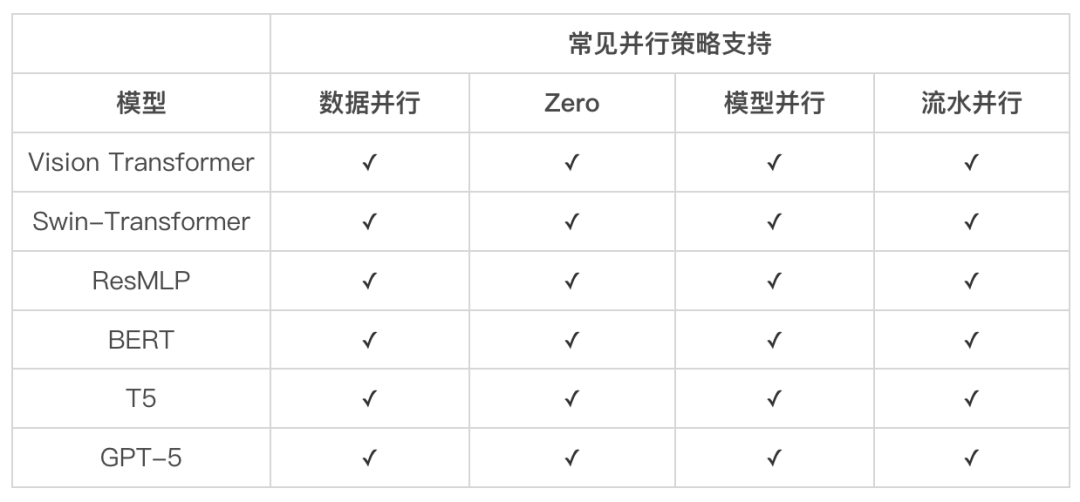LiBai 目前已支援 BERT、GPT、ViT、Swin-Transformer、T5 等常見模型，以及 MoCoV3、MAE 等最新研究，開箱即用，並且可以很方便地在下游任務上進行微調。

• LiBai 模型庫地址：https://github.com/Oneflow-Inc/libai